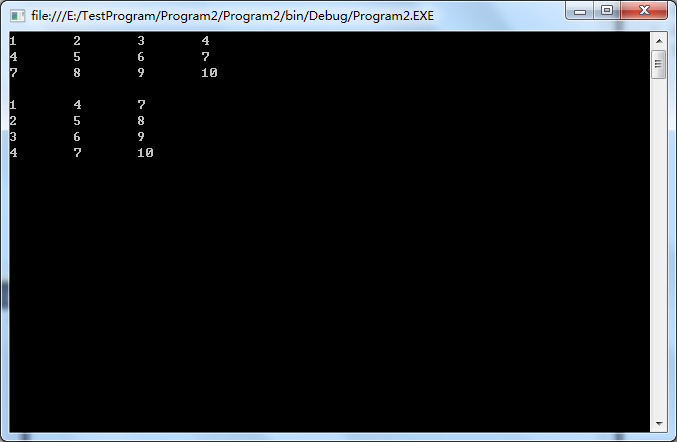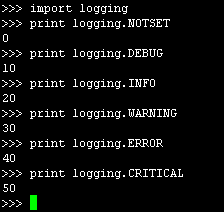• matlab中实现矩阵删除一行或一列的方法实例如下所示：>> A=[1,2,3;4,5,6;7,8,9]A =1 2 34 5 67 8 9删除行：>> A(2,:)=[]A =1 2 37 8 9删除列：>> A(:,2)=[]A =1 37 9以上这篇matlab中实现矩阵删除...

matlab中实现矩阵删除一行或一列的方法

实例如下所示：

>> A=[1,2,3;4,5,6;7,8,9]

A =

1 2 3

4 5 6

7 8 9

删除行：

>> A(2,:)=[]

A =

1 2 3

7 8 9

删除列：

>> A(:,2)=[]

A =

1 3

7 9

以上这篇matlab中实现矩阵删除一行或一列的方法就是小编分享给大家的全部内容了，希望能给大家一个参考，也希望大家多多支持我们。

您可能感兴趣的文章:

Python numpy 提取矩阵的某一行或某一列的实例

Python矩阵常见运算操作实例总结

Python表示矩阵的方法分析

Python获取二维矩阵每列最大值的方法

Python实现矩阵转置的方法分析

python中返回矩阵的行列方法

时间： 2018-04-01

本文实例讲述了Python矩阵常见运算操作.分享给大家供大家参考,具体如下: python的numpy库提供矩阵运算的功能,因此我们在需要矩阵运算的时候,需要导入numpy的包. 一.numpy的导入和使用 from numpy import *;#导入numpy的库函数 import numpy as np; #这个方式使用numpy的函数时,需要以np.开头. 二.矩阵的创建 由一维或二维数据创建矩阵 from numpy import *; a1=array([1,2,3]); a1=ma本文实例讲述了Python表示矩阵的方法.分享给大家供大家参考,具体如下: 在c语言中,表示个"整型3行4列"的矩阵,可以这样声明:int  a;在python中一不能声明变量int,二不能列出维数.可以利用列表中夹带列表形式表示.例如: 表示矩阵 ,可以这样: count = 1 a = [] for i in range(0, 3): tmp = [] for j in range(0, 3): tmp.append(count) count += 1 a.append

因为做项目中间有一个很小的环节需要这个功能,所以就写了一个简单的小函数,下面是具体实现: #!usr/bin/env python #encoding:utf-8 ''' __Author__:沂水寒城 ''' def get_max_value(martix): ''' 得到矩阵中每一列最大的值 ''' res_list=[] for j in range(len(martix)): one_list=[] for i in range(len(martix)): one_list.ap

实例如下所示: # TODO 返回矩阵的行数和列数 def shape(M): return len(M),len(M) 以上这篇python中返回矩阵的行列方法就是小编分享给大家的全部内容了,希望能给大家一个参考,也希望大家多多支持我们. 您可能感兴趣的文章: Python numpy 提取矩阵的某一行或某一列的实例 Python矩阵常见运算操作实例总结 Python表示矩阵的方法分析 Python获取二维矩阵每列最大值的方法 Python实现矩阵转置的方法分析 matlab中实现矩阵删

如下所示: import numpy as np a=np.arange(9).reshape(3,3) a Out: array([[0, 1, 2], [3, 4, 5], [6, 7, 8]]) 矩阵的某一行 a Out: array([3, 4, 5]) 矩阵的某一列 a[:,1] Out: array([1, 4, 7]) b=np.eye(3,3) b Out: array([[ 1., 0., 0.], [ 0., 1., 0.], [ 0.,本文实例讲述了Python实现矩阵转置的方法.分享给大家供大家参考,具体如下: 前几天群里有同学提出了一个问题:手头现在有个列表,列表里面两个元素,比如[1, 2],之后不断的添加新的列表,往原来相应位置添加.例如添加[3, 4]使原列表扩充为[[1, 3], [2, 4]],再添加[5, 6]扩充为[[1, 3, 5], [2, 4, 6]]等等. 其实不动脑筋的话,用个二重循环很容易写出来: def trans(m): a = [[] for i in m] for i in m: f

本文实例讲述了python实现矩阵乘法的方法.分享给大家供大家参考.具体实现方法如下: def matrixMul(A, B): res = [ * len(B) for i in range(len(A))] for i in range(len(A)): for j in range(len(B)): for k in range(len(B)): res[i][j] += A[i][k] * B[k][j] return res def matrixMul2(A, B):本文实例讲述了C#实现矩阵转置的方法.分享给大家供大家参考.具体如下: 1.转置函数 /// /// 求一个矩阵的转置矩阵 /// /// 矩阵 /// 转置矩阵 private static double[][] MatrixTranspose(double[][] matri

本文实例讲述了Windows平台Python连接sqlite3数据库的方法.分享给大家供大家参考,具体如下: 之前没有接触过sqlite数据库,只是听到同事聊起这个. 有一次,手机端同事让我帮着写个sql,后面说运行不了报错了,我问是什么数据库,同事说是sqlite,这才知道了还有sqlite这个数据库... 接下来说说Python连接sqlite数据库,非常简单,因为python中的sqlite模块也遵循了DB-API 2.0的规范,所以操作起来和sql server.MySQL.oracle

本文实例讲述了Python自定义线程池实现方法.分享给大家供大家参考,具体如下: 关于python的多线程,由与GIL的存在被广大群主所诟病,说python的多线程不是真正的多线程.但多线程处理IO密集的任务效率还是可以杠杠的. 我实现的这个线程池其实是根据银角的思路来实现的. 主要思路: 任务获取和执行: 1.任务加入队列,等待线程来获取并执行. 2.按需生成线程,每个线程循环取任务. 线程销毁: 1.获取任务是终止符时,线程停止. 2.线程池close()时,向任务队列加入和已生成线程等量的本文实例讲述了基于Python开发chrome插件的方法.分享给大家供大家参考,具体如下: 谷歌Chrome插件是使用HTML.JavaScript和CSS编写的.如果你之前从来没有写过Chrome插件,我建议你读一下这个.在这篇教程中,我们将教你如何使用Python代替JavaScript. 创建一个谷歌Chrome插件 首先,我们必须创建一个清单文件:manifest.json. { "manifest_version": 2, "name": "Py本文实例讲述了python日志logging模块使用方法.分享给大家供大家参考,具体如下: 一.从一个使用场景开始 开发一个日志系统, 既要把日志输出到控制台, 还要写入日志文件 import logging # 创建一个logger logger = logging.getLogger('mylogger') logger.setLevel(logging.DEBUG) # 创建一个handler,用于写入日志文件 fh = logging.FileHandler('test.log') fh

本文实例讲述了python装饰器常见使用方法.分享给大家供大家参考,具体如下: python 的装饰器,可以用来实现,类似spring AOP 类似的功能.一样可以用来记录某个方法执行前做什么,执行后做什么,或者用来记录日志,运行的时间等,更有甚者,用这个来做权限拦截,也未尝不可.从两个方面来描述python 的装饰模式: 1. 对普通方法的装饰 2. 对在 class 类中的方法的装饰,不需要给参数的情况 3. 对在 class 类中的方法的装饰,需要给参数的情况 一,对普通方法的装饰.比如,

本文实例讲述了Python实现购物车功能的方法.分享给大家供大家参考,具体如下: 1.程序的源代码如下: salary = input('input your salary:') if salary.isdigit: salary = int(salary) else: exit('salary is not digit!!') welcome_msg = 'welcome to our shoping mall' print(welcome_msg.center(50,'-')) produc

本文实例讲述了Python实现识别图片内容的方法.分享给大家供大家参考,具体如下: python识别图片内容. 这里我的环境为windows64位,python2.7.14 需要用到PIL模块和tesseract模块. 首先需要安装pip包管理,安装方法可参考附录windows下安装python包管理器pip 安装PIL模块: pip install Pillow tesseract模块安装: pip install pytesseract 安装识别引擎和中文语言包,点击此处本站下载. 下载完成

展开全文• 我想让矩阵A的第四列所有小于26.5的数值删除整行，请问该怎么做呢？loop i的次数好像不能跟着矩阵的缩小而变化。matlab 开发语言
• deleteRC.m 自己写的代码，还算好用，可以次性删除任意或列的数据 删除矩阵X中index指定的或列，type='r'表示，type='c'表示列
• 遍历所有，找到满足要求的tag，然后调用A(a,:) = []。 A = neighborhood_s; a = []; for i = 1 : size(A,1) if abs(A(i,7)) > 1.0 || abs(A(i,8)) > 1.0 a = [a; i]; end end A(a, :) = []; 耗时20...

方法1

遍历所有行，找到满足要求的行tag，然后调用A(a,:) = []

A = neighborhood_s;
a = [];
for i = 1 : size(A,1)
if abs(A(i,7)) > 1.0 || abs(A(i,8)) > 1.0
a = [a; i];
end
end

A(a, :) = [];

耗时20分钟！

方法2

使用逻辑判断，找到行tag，然后调用A(id,:) = []

注意是|，如果使用||会报错“|| 和 && 运算符的操作数必须能够转换为逻辑标量值”。

id = abs(A(:,7)) > 1.0 | abs(A(:,8)) > 1.0;
A(id,:) = [];

耗时2秒！

方法3

使用find函数，找到行tag，然后调用A(id,:) = []

id = find(abs(A(:,7)) > 1.0 | abs(A(:,8)) > 1.0);
A(id,:) = [];

耗时2秒！

总结

上述三种方法中：方法3耗时最短，为2秒；方法1耗时最长，为20分钟；方法2和方法3的耗时在同一量级上。推荐使用方法2和方法3。

展开全文matlab 笔记
• 输入一个矩阵A >> A = [1, 2, 3; 4, 5, 6; 7, 8, 9] A = 1 2 3 ...删除一行 >> A(2, :) = [] A = 1 2 3 7 8 9 输入一个矩阵A >> A = [1, 2, 3; 4, 5, 6; 7, 8, 9] A = 1 2

输入一个矩阵A

>> A = [1, 2, 3; 4, 5, 6; 7, 8, 9]

A =

1     2     3
4     5     6
7     8     9

删除一行

>> A(2, :) = []

A =

1     2     3
7     8     9

输入一个矩阵A

>> A = [1, 2, 3; 4, 5, 6; 7, 8, 9]

A =

1     2     3
4     5     6
7     8     9

删除一列

>> A(:, 2) = []

A =

1     3
4     6
7     9

欢迎访问我的个人网站：

李培冠博客：lpgit.com

展开全文matlab
•     在Matlab2016中，输入矩阵A=[1 2 3;... 若要删除一行的元素则A(1,:)=[] <textarea readonly="readonly" name="code" class="c++"> >> A=[1 2 3;4 5 6;7 8 9]; &...

在Matlab2016中，输入矩阵A=[1 2 3;4 5 6;7 8 9];只取矩阵中第一行的元素A1=A(1,:); 若要删除第一行的元素则A(1,:)=[]

>> A=[1 2 3;4 5 6;7 8 9];
>> A1=A(1,:)

A1 =

1     2     3

>> A(1,:)=[]

A =

4     5     6
7     8     9
</textarea>

如果要删除矩阵A中后面两行，则有
A=[1 2 3;4 5 6;7 8 9];
A(2:3,:)=[]

A=[1 2 3;4 5 6;7 8 9];
A(2:3,:)=[]

A =

1     2     3
</textarea>

如果要删除矩阵A中后面两列，则有
A=[1 2 3;4 5 6;7 8 9];
A(:,2:3)=[]

A=[1 2 3;4 5 6;7 8 9];
A(:,2:3)=[]

A =

1
4
7
</textarea>

展开全文matlab
• matlab中矩阵删除一行或一列的方法

万次阅读 多人点赞 2017-11-23 22:06:54matlab
• 删除第2 a = [1 1; 2 2; 3 3; 4 4]; a(2,:) = []; 得到：a = [1 1; 3 3; 4 4] 2，不知道行号，删除指定的元素 a = [1 1; 2 2; 3 3; 4 4; 2 2]; b = [3 3]; c = setdiff(a,b,'row','stable'); 得到：c = [1 1; 2 ...matlab setdiff
• 矩阵中去除某一行/列[matlab]

万次阅读 2017-11-30 20:05:05
去掉第n列：  S(:,n) = []; 去掉第m： S(m,:)=[];matlab 矩阵行列
• 有一个矩阵, 举例如下: A = 6 11 17 1 1 1 3 5 6 6 11 15 1 3 6 ...目前网上的教程都是如何删除元素相同的行, 那么如果我们想删除元素相同的一行, 比如1 1 1该怎么办呢? 我们可以换个思路: 把
• Matlab删除矩阵的某行某列形成新矩阵的操作方法操作方法：通过空矩阵转换删除打开Matlab的命令行窗口，输入“A=[ 1 2 3;4 5 6;7 8 9]”，按回车键，输入“B=A;B(:,)=[];B”代码，这是删除A矩阵的第列...
• MATLAB删除矩阵一列或某一行删除某一行例子删除某一列例子 删除某一行 b([列数],:) = []; // b为矩阵名 例子 删除一行 删除第三行 删除某一列 b(:,[列数]) = []; // b为矩阵名 例子 删除第...matlab
• matlab删除矩阵中的某些行

千次阅读 2020-03-19 20:57:24
A=[1 2 3 4 5 6 7 8 9 10 11 12]; A(5,:)=[];A(3,:)=[];%一定要从后往前删除，否则会出错 计算结果： A = 1 2 3 4 7 8 11 12 ...矩阵
• G=[1,2,3;4,5,6;7,8,9];删除第三G(3,:) = []; 删除第3列G(:,3) = [];MATLAB
• MATLAB删除矩阵全零行

千次阅读 2020-08-20 15:11:05
**vector = all(array, 2)**返回一个列向量，用于检测每一行是否全为非零元素，如果某一行中有一个元素为零，则返回0，如果某一行中全为非零元素，则返回1，由此构成一个列向量。 例子 删除全零行 >> a = [1,...matlab
• 1、打开matlab，在命令行窗口中...两种方法：1，将所有要删除标顺序排列成向量V，然后用命令举个例子，思路就是利用逻辑运算，找到符合条件的，然后新的矩阵只取不满足条件的那几行：>&"矩阵变量名"(V,...
• 在用matlab编程进行GNSS粗差提取时，想实现对某矩阵删除指定行（指定列）的命令，下文中描述的均为行。通过百度，先明白对于一行数据而言，删除指定位置的元素的原理。主要思路是先提取要删除的位置，再对该位置数据...matlab
• 近来用matlab编程遇到了这样两个问题，如有以下矩阵：IDX = [1,2,3;2,3,1;1,2,3;2,3,1;1,1,1;1,1,1];问题1)怎样去掉其中的重复呢？在网上找了半天，大家都提供的是自己写的循环。我在想，这样简单的问题matlab肯定...
• 注意个参数表示时，MATLAB是列优先的，千万别弄错。例如：A=[1,2,3;4,5,6;7,8,9];则A(2，1)=4。千万别写成A[2,1]!如果用维表示是：A(2)=4,千万别写成A(4)!不信你可以上机试试www.mh456.com防采集。解决这个问题...
• 0 9 7]这是一个3*3矩阵，那么当你想插入一行r=[1 2 3]时，那么可以这样做：A=[A;r]这样A就变成了4*3的矩阵A如下：A=[2 3 5;3 4 1;0 9 7;1 2 3]假如你已有矩阵A为：A=[2 3 5;3 4 1;0 9 7;1 2 3]那...答：用A...
• MATLAB删除矩阵元素

千次阅读 2020-06-05 19:06:56matlab
• Python numpy 提取矩阵某一行一列的实例如下所示：import numpy as npa=np.arange(9).reshape(3,3)aOut:array([[0, 1, 2],[3, 4, 5],[6, 7, 8]])矩阵某一行aOut: array([3, 4, 5])矩阵一列a...
• matlab矩阵去掉重复行

千次阅读 2021-07-19 11:06:10
比如我的每个解还对应着他的两个目标值，那你可能还需要返回的下标，用于处理关联矩阵，如下 [uniqueA row]=unique(A,'row','stable') 再者，你如果有从去重后的矩阵回复原矩阵的想法，那你可能还需要另个...
• matlab去除矩阵某几行的值，并且保持其余元素顺序不变matlab matlab
• 试试这个衬垫(在哪一是你的交集矩阵和乙是要删除的值):A = [-4.0 0.5;-2.0 0.5;2.0 3.0;...然后对每一个新的重复最后一行乙您要删除。编辑:…还有另一个选择:A = A((A(:,1) ~= B(1)) | (A(:,2) ...
• reshape(x,m,n)%%x为向量，m*n=length(x)>> a=rand(1,16);>> b=reshape(a,2,8)b =0.4447 0.7919 0.7382 0.4057 0.9169 0.8936 0.3529 0.00990.6154 0.9218 0.1763 0.9355 0.4103 0.0579 0.8132 0.1389...
• Matlab矩阵

2021-04-20 12:26:44
Matlab 的 Matlab矩阵矩阵是数字的二维数组。在MATLAB中，可以通过在每行中输入元素来创建一个矩形，以逗号或空格分隔数字，并使用分号标记每一行的结尾。例如，创建一个4×5矩阵a -a = [ 1 2 3 4 5; 2 3 4 5 6; 3 4......

matlab矩阵删除某一行matlab 订阅